[BACK]
 Computers, Materials & ContinuaDOI:10.32604/cmc.2021.015154Article

Deep Learning and Improved Particle Swarm Optimization Based Multimodal Brain Tumor Classification

1Department of Computer Science, HITEC University, Taxila, 47040, Pakistan
2College of Computer Science and Engineering, University of Ha’il, Ha’il, Saudi Arabia
3Department of Computer Science and Engineering, Soonchunhyang University, Asan, Korea
4School of Architecture Building and Civil Engineering, Loughborough University, Loughborough, LE11 3TU, UK
5Department of Robotics, SMME NUST, Islamabad, Pakistan
*Corresponding Author: Yunyoung Nam. Email: ynam@sch.ac.kr
Received: 08 November 2020; Accepted: 05 February 2021

Abstract: Background: A brain tumor reflects abnormal cell growth. Challenges: Surgery, radiation therapy, and chemotherapy are used to treat brain tumors, but these procedures are painful and costly. Magnetic resonance imaging (MRI) is a non-invasive modality for diagnosing tumors, but scans must be interpretated by an expert radiologist. Methodology: We used deep learning and improved particle swarm optimization (IPSO) to automate brain tumor classification. MRI scan contrast is enhanced by ant colony optimization (ACO); the scans are then used to further train a pretrained deep learning model, via transfer learning (TL), and to extract features from two dense layers. We fused the features of both layers into a single, more informative vector. An IPSO algorithm selected the optimal features, which were classified using a support vector machine. Results: We analyzed high- and low-grade glioma images from the BRATS 2018 dataset; the identification accuracies were 99.9% and 99.3%, respectively. Impact: The accuracy of our method is significantly higher than existing techniques; thus, it will help radiologists to make diagnoses, by providing a “second opinion.”

Keywords: Brain tumor; contrast enhancement; deep learning; feature selection; classification

1  Introduction

Brain tumors are the 10th most common type of cancer worldwide [1,2], and glioma is the most prevalent brain tumor. A low-grade glioma (LGG) can be cured if diagnosed early; high-grade gliomas (HGGs) are malignant. Generally, an LGG does not spread . The World Health Organization grades benign and malignant tumors as I, II and III, IV, respectively . Symptoms include difficulty speaking, short-term memory loss, frequent headaches, blurred vision, and seizures; these vary by tumor size and location. Magnetic resonance imaging (MRI) is used to visualize brain tumors. However, accurate classification is not possible with a single MRI sequence; multiple MRI sequences (T1, T1 with contrast enhancement, T2, and FLAIR  are required). In the United States alone, approximately 22,850 patients are diagnosed with brain tumors annually ; the number in 2019 was 23,890 (13,590 males and 10,300 females), including 18,020 deaths (10,190 males and 7,830 females). MRI is much more efficient than computed tomography; the amount of radiation is lower, while the contrast is higher. Analysis of MRI scans is difficult ; an automated approach is required . The typical analytical steps include preprocessing, feature extraction and reduction, and classification. Some researchers have used image segmentation for tumor detection, while others have focused on feature extraction for classification based on tumor intensity and shape [8,9]. Features extraction is an essential step in disease classification . Based on the features, the tumor is identified by feature properties including intensity, shape, etc. More recently, deep learning gives more impressive results for medical infection classification. Deep learning is invaluable for detecting and classifying tumors . There are several pretrained models  that classify extracted features using supervised learning algorithms such as Softmax, support vector machine (SVM), naïve Bayes, and K-nearest neighbor (KNN) .

In medical imaging, deep learning shows huge performance for both disease detections and classification. The major medical diseases are brain tumors , skin cancers , lung cancers , stomach conditions , retinal injuries , and blood diseases , among other conditions . Brain tumor analysis remains challenging ; several techniques are available but none of them are 100% accurate [23,24]. Most techniques are based on machine learning , which facilitates early tumor detection . Convolutional neural networks (CNNs) , K-means algorithms , decision-level fusion , machine learning-based evaluation , and deep learning  approaches have all been used. Tanzila et al.  accurately detected tumors using feature fusion and deep learning. A grab-cut method was used for segmentation. The geometry of a transfer learning (TL) model was fine-tuned to identify features, and a serial-based method was used to fuse them. All features were optimized by entropy. The tumor detection accuracy was 98.78% for BRATS 2015, 99.63% for BRATS 2016, and 99.67% for BRATS. Schadeva et al.  improved segmentation and brain tumor classification accuracy using an active contour model that focused on the area of interest; features were extracted, reduced by principal component analysis, and classified using an automated neural network. The classification accuracy was 91%. Mohsen et al.  used deep learning for brain tumor classification. MRI scans were segmented using the fuzzy c-means approach and discrete wavelet transformation was applied to extract features. A deep neural network performed the classification with an accuracy of 96.97%. The linear discriminant analysis (LDA) accuracy was 95.45% and that of sequential minimal optimization (SMO) was 93.94%. The deep learning network resembled a CNN, but required less hardware and was much faster.

Problem Statement: The major challenges in brain tumor classification are as follows: (i) manual evaluation is difficult and time-consuming; (ii) tumor resolution is low and irrelevant features may be highlighted; (iii) redundant features cause classification errors; and; (iv) tumors grades I–IV look relatively similar. To resolve these issues, we present an automated classification method using deep learning and an improved particle swarm optimization (IPSO) algorithm.

Contributions: The major contributions of this study are as follows: (i) MRI scan contrast is improved using an evolutionary approach, i.e., ant colony optimization (ACO); (ii) a pretrained VGG-19 model is fine-tuned via TL; (iii) features are extracted from two different dense layers and fused into one matrix; and, (iv) the IPSO is combined with a bisection method for optimal feature selection.

The remainder of this manuscript is organized as follows. The ACO, improvement of the original image contrast, TL -based fine-tuning, serial feature fusion, and IPSO are discussed in Section 2, the HGG and LGG results are presented in Section 3, and the conclusions are provided in Section 4.

2  Proposed Methodology

We used deep learning for multimodal classification of brain tumors. The contrast of the original images was improved by ACO, and the images were used to train a CNN. TL of brain images was used to enhance a pretrained model. Features computed by different layers were aggregated, and the IPSO was used to select optimal features that were then classified using a one-against-all multiclass SVM (MSVM) classifier. The overall architecture is shown in Fig. 1.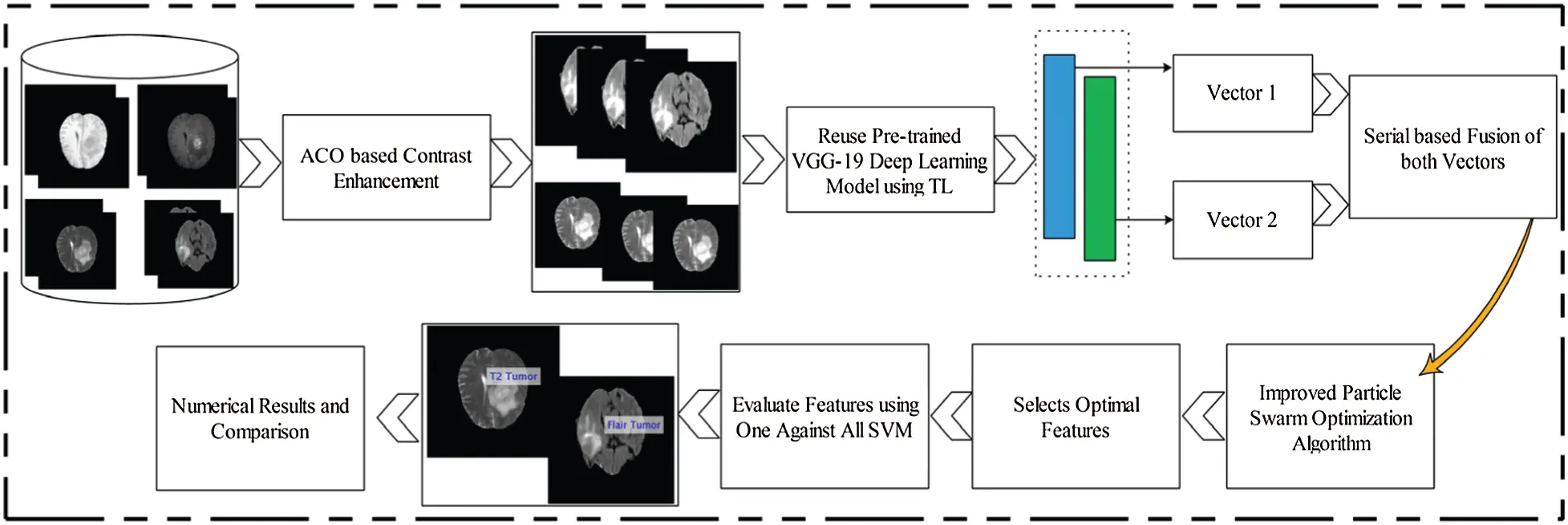Figure 1: Proposed architecture diagram of multimodal brain tumor classification using deep learning

2.1 Contrast Enhancement

Contrast enhancement is very important because unenhanced images exhibit low contrast, noise, and very poor illumination . Several enhancement techniques are available; we used an ACO-based approach.

Initial Ant Distribution—The number of ants is calculated as:

AN=l×w(1)

where l is the length of the image, w is the width, and AN is the number of ants randomly placed in the image (one pixel=one ant).

Decision-based on Probability—The probability that ant n moves from pixel (e, f) to pixel (g, h) is given by:

Pef=(ρef)a(ωef)buef(Δ) fQ(ρef)a(ωef)buef(Δ)(2)

When e,fΩ

Here, all pixel locations are written e,fΩ. ρef is the pheromone level. ωef the visibility, and is calculated as follows:

ωef=Hef(3)

The probability equation shows that Δ-plus reflects the stepwise directional fluctuation:

Δ=0,π/4,π/2,3π/4,π(4)

where u(Δ) is the weight function. Together with the function above, the weight function ensures that sharp turns by ants are less frequent than gentle ones, which we refer to as “probabilistic forward bias.”

Rule of Transition—Mathematically, the rule of transition is expressed as:

s={arg{maxjQ[(ρij)a(ωij)buij(Δ)]}},whenq<q(5)

where ij is the pixel location, from which ants can travel to pixel (k,l). If q>q, an ant can visit the next pixel [see Eq. (2)].

Updating Pheromone Levels—An ant can move from pixel ij to pixel (k,l), as stated above, and the pheromone trajectory is given by:

ρij=(1-η).ρij+η.Δρij (6)

Δρij=ωij (7)

A new trajectory is obtained after each iteration, as follows:

ρij=(1-Θ).ρij+Θ.ρ(8)

where Θ(0<Θ<1) is the proportion of pheromone that evaporates and ρ is the initial pheromone concentration . Applying the above steps to all image pixels yields an enhanced image (Fig. 2).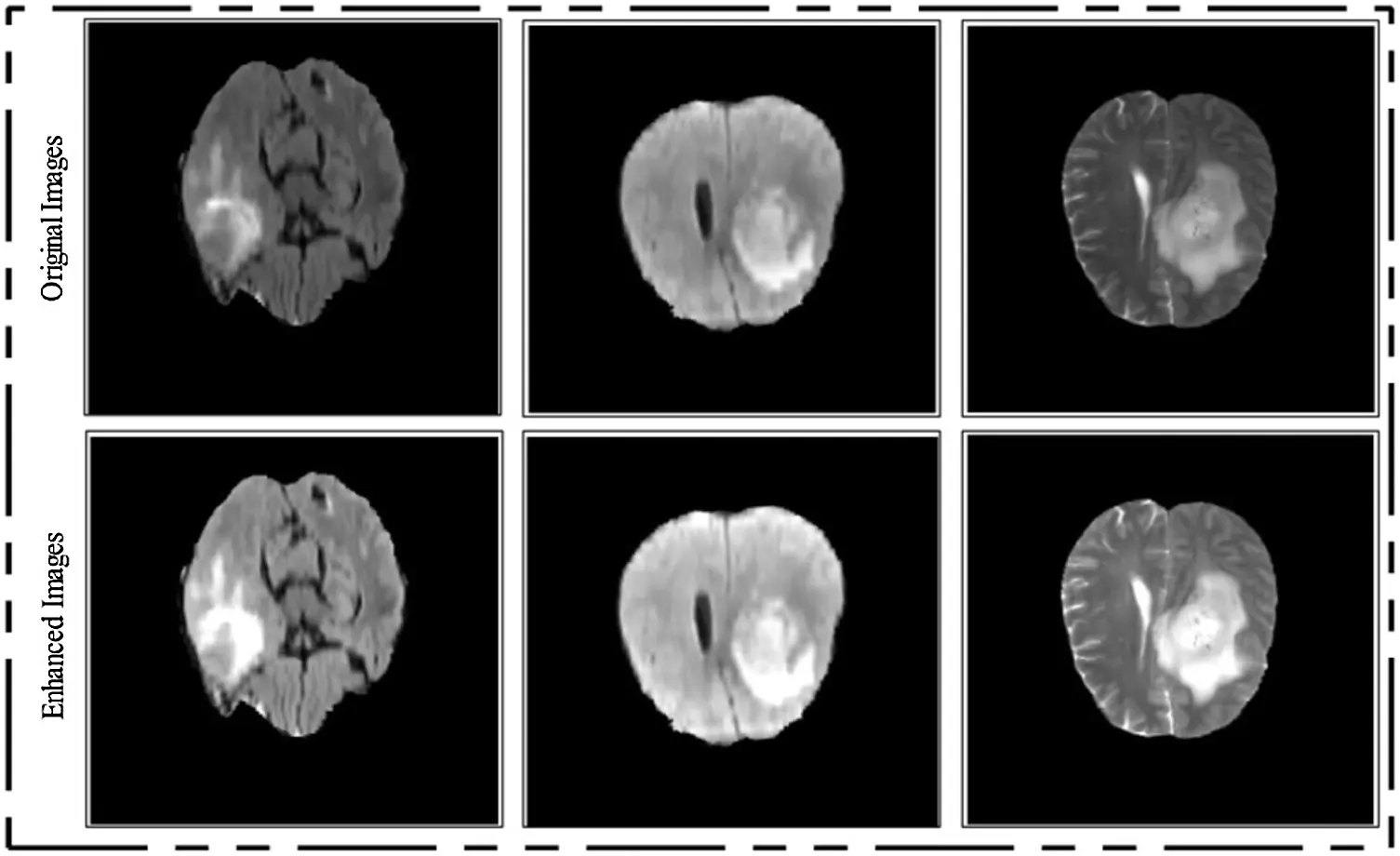Figure 2: Visual description of contrast stretching results on original images

2.2 Convolutional Neural Network

A CNN is a type of deep neural network that can be used for image recognition and classification, and object detection . A CNN requires minimal preprocessing. During training and testing, images pass through kernel layers, and are pooled and then fully connected; this is followed by Softmax classification. Probability values range from 0 to 1. Several pretrained CNN models are available, including VggNet and AlexNet . VggNet has valuable medical applications . We used a pretrained VGG-19 model  which includes 16 convolutional layers (local features), 3 fully connected layers, and max-pooling and ReLu layers (Fig. 3).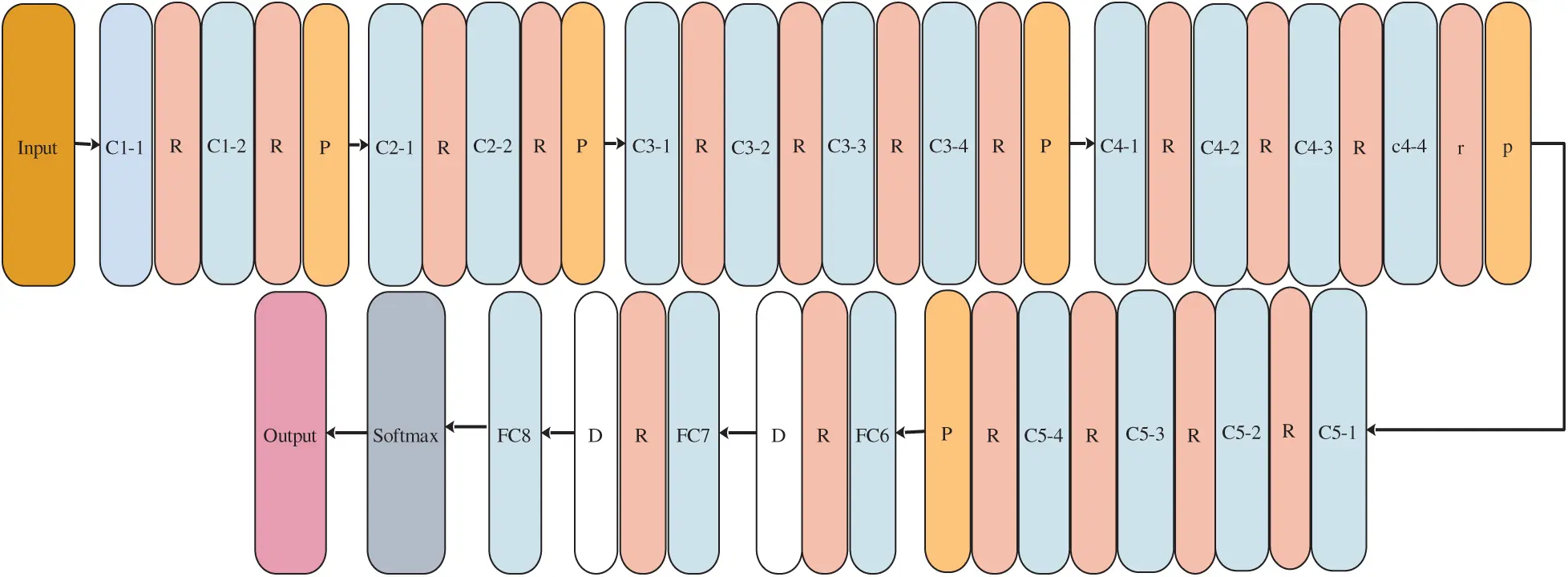Figure 3: VGG-19 architecture

2.3 VGG-19

VGG-19 contains N fully connected layers, where N = 1–3. The PN units of the Nth layers are NRW = 224, Nc = 224 and Nch = 3. The dataset is represented by α, and the training sample by Wabϵα. Each Wab is a real number R:

ω(1)=r(n(1)Wab+γ(1))ϵR(1)(9)

where ω(1) is the first weight matrix, r() is the Relu activation function, RW the number of rows, c the number of columns, and ch the number of channels. γ(1) is the bias vector and n(1) is the weight of the first layer, defined as:

n(1)ϵRN(1)×q(10)

The output of the first layer becomes the input of the second layer; this step is repeated as follows:

ω(2)=r(n(2)ω(1)+γ(2))ϵR(2) (11)

ω(3)=r(n(3)ω(2)+γ(3))ϵR(3) (12)

ω(4)=r(n(4)ω(3)+γ(4))ϵR(4) (13)

ω(5)=r(n(5)ω(4)+γ(5))ϵR(5) (14)

Here, by way of example, ω(2) and ω(3) are the second and third weight matrices, respectively. n(2)ϵRN(2)×N(1) and n(2)ϵRN(2)×N(1). ω(Z) represents the last fully connected layer used for high-level feature extraction. Mathematically:

ωh(Wab)=ω(19)=r(n(19)ω(18)+γ(19))ϵR(19) (15)

A(e)=cl=1MB(0b,cl) log(p(0b,cl)) (16)

where A(e) is the cross-entropy function, B is the total number of classes cl, and ob and p the predicted probabilities.

2.4 Transfer Learning

TL occurs when a system acquires knowledge and skills by solving a specific problem, and then uses that knowledge to solve another problem . We used TL to further train, and improve the performance, of a pretrained model. The input was Ip={(a1p,b1p),,(ai,pbip),,(anp,bnp)}, and the original learning task can be described as: ld,lp,(am,pbmp)ϵR. The target was To={(a1o,b1o),,(ai,obio),,(amo,bmo)}; and the new learning task can be written as lt,(ano,bnoϵR,(m,n), where n < < m and b1Iandb1o are the training data labels (Fig. 4).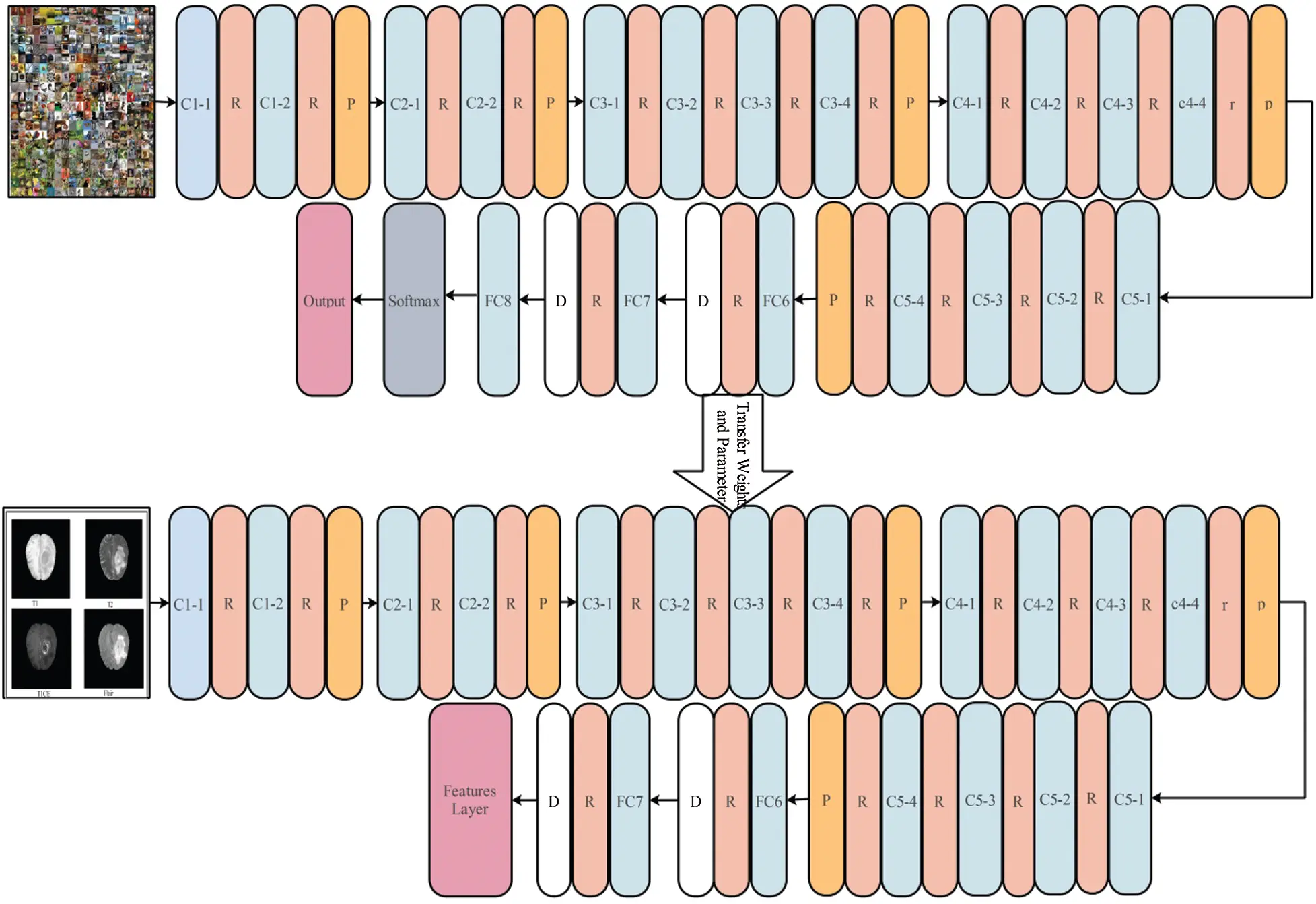Figure 4: Transfer learning based retraining a model for multimodal brain tumor classification

Feature Extraction and Fusion: After TL, activation is required for feature extraction. We extracted features from FC layers 6 and 7. The feature vector of FC layer 6 had dimensions of N×4,096, and that of FC layer 7 4,096. Mathematically, the vectors are expressed as FVk1N and FVk2N; both FVk1N and FVk2N. We then fused the vectors into a single matrix to derive optimal tumor data. This can be done using serial, parallel, and correlational techniques. We used the lengths of extracted features and no features were discarded. Mathematically, the fused matrix can be expressed as:

FVk3N=(FVk1NFVk2N)N×(k1+k2)(17)

where FVk3N is a fused matrix with dimensions of k1×k2. N is the number of images used for training and testing. k1 and k2 both have a value of 4,096. The fused vector includes a few irrelevant/redundant features, which were removed by IPSO.

2.5 Features Selection and Classification

It is important to select appropriate features for classification, because irrelevant features reduce classification accuracy and increase the computational time . However, it is not easy to identify the most important features because of their complex interactions. A good feature vector is required; in this study we used the IPSO algorithm. The original PSO  was a global search algorithm using evolutionary computation. PSO, as a population-based algorithm inspired by flocks of birds and schools of fish, is more effective than a general algorithm  in terms of convergence speed. Particles are initially placed randomly, and their velocities and positions are iteratively updated. The current and updated particle locations are referred to as pbest and gbest, respectively. The IPSO reduces the number of iterations required by including a “stop” condition based on a bisection method (BsM). The selected values are approximated and the algorithm is then terminated; the accuracy of each iteration is approximately the same as the previous one. Assuming that the position of the nth particle is Yi=yi1,yi2,,yiM and the velocity is Vi=Vi1,Vi2,,ViM, the local best particle is Li=li1,li2,,lin and the global best particle is Gb=gb1,gb2,,gbM. The updated position of the ith particle is calculated as:

Vij(s+1)=x.Vij(s)+a1.R1.(lim(s)-yim(s))+a2.R2.(gbm(s)-yim(s)) (18)

yim(s+1)=yim(s)+Vij(s+1) (19)

where -=1,2,3,,N, m=1,2,3,M, S is the number of iterations, N is the size of the swarm, R1 and R2 are random numbers [0, 1], a1anda2 are acceleration coefficients, and x is the inertial weight. A linear value of x that varies with time is calculated as:

x(s)=xmax=xmax-xminT.s(20)

Here, T is the maximum iteration time, xmax is the upper limit, and xmin is the lower limit. During feature selection, every solution is a subset of features. Each set of particles is denoted as a binary vector, and every particle has a specific position. The Mth feature is defined by the Mth position. Features are selected by the IPSO, which begins with a random solution and then moves toward the best global solution (represented by a new subset of features). Each feature is linked to a dataset that occupies a search space. If the Mth position is 1, the Mth feature is considered informative, while if the Mth position is 0, the Mth feature is not informative. If the Mthposition is −1, the Mth feature is not added to the set.

Fitness Function: Each solution yielded by the selection algorithm was tested in terms of fitness within every generation. If accuracy improved, the current solution was the best one. The solution with maximum fitness is the best one overall. We used the fine KNN classifier and BsM. The starting accuracy was 90.0 (t̃), and the final accuracy is expressed as t. The midpoint of t̃ and t was computed and the root was found. If the root was equal to zero, the algorithm terminated; otherwise, the next iteration started and the root between t and t + 1 was found. If the interval was not zero, the midpoint of t and t + 1 was determined, and the following criteria were checked:

Criteria={iff(mid)×f(t+1)<0thenUpdatet=midElsewheret+1=mid(21)

Thus, the values were updated until two successive iterations became very similar. We initially selected 100 iterations, but the algorithm stopped between 10 and 20 iterations, yielding a N×1,875 vector containing approximately 40% of all features that were finally classified using the one-against-all SVM.

Consider an N-class problem with B training samples, (s1,t1),,(sn,tn), where siϵRa is an n-dimensional feature vector and tiϵ{1,2,,N}. The method builds N binary SVM classifiers, and each classifier separates all classes. Training of the i-th SVM uses all samples with ith-positive labels and the remaining negative labels di(S)=xipØ(S)+ei:

MinimizeK(x.ji=12xi2+Fl=1nji (22)

Subjectto:t,j(xipØ(sj)+ei)1-ji,ji0 (23)

t,j=1 if tj = i, and t,j=-1 otherwise.

Sample s is classified into the class i*, the d* of which is the highest during classification:

i*= argmaxdi(s)= argmax(xipØ(s)+ei),i=1,2,,Ai=1,2,,(24)

3  Experimental Results and Comparison

We analyzed the BRATS 2018 dataset , which contains HGG and LGG data. In total, 70% of the data were used for training and 30% for testing (Fig. 5). We evaluated multiple classifiers in terms of accuracy, sensitivity, precision, the F1-score, the area under the curve (AUC) the false-positive rate (FPR), and computational time. All simulations were run on Matlab 2019a (MathWorks, Natick, MA, USA) using a Core i7 processor, 16 GB of RAM, and an 8 GB graphics card.Figure 5: Proposed testing process

3.1 Testing Results of HGG Images Data

We first classified HGG images (30% of all test images). The results obtained via fusion of the original feature vectors are shown in Tab. 1. The highest accuracy was 99.9%, for the MSVM, with a sensitivity of 99.25%, precision of 99.50%, F1-score of 99.3%, FPR of 0.00, and AUC of 1.00. The other accuracies were as follows: fine tree, 89.20%; linear SVM, 98.70%; coarse Gaussian, 95.80%; fine KNN, 99.70%; medium KNN, 97.70%; cubic KNN, 97.0%; weighted KNN, 99.20%; ensemble-boosted tree, 96.40%; and ensemble-bagged tree, 98.0%. Thus, the MSVM performed best. The confusion matrix is shown in Fig. 6; the accuracy rate always exceeded 99%. The computational times are listed in Tab. 1. The medium KNN had the shortest computational time, at 28.52 s but the accuracy was only 97.75%. The receiver operator characteristic (ROC) curves are shown in Fig. 7.

Table 1: Classification results for the proposed method using original fused feature vectors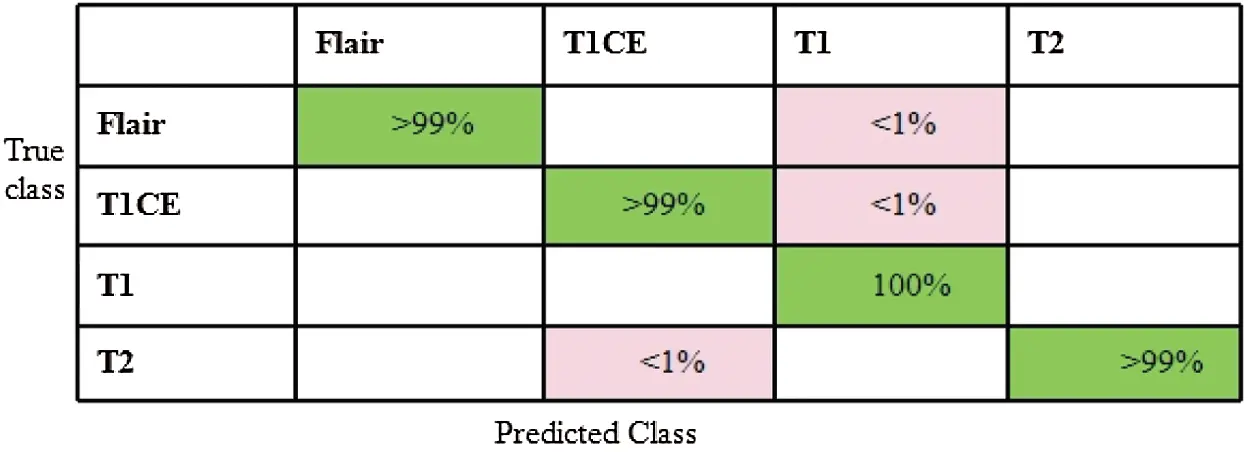Figure 6: Confusion matrix for MSVM using original fused feature vectorsFigure 7: ROC plots of MSVM using original fused feature vectors

The optimized HGG features are listed in Tab. 2 (HGG). The highest accuracy was 99.9%, for the MVSM, followed by 85.20% for the fine tree classifier, 98.75% for the linear SVM, 95.50% for the course Gaussian, 99.60% for the fine KNN, 97.30% for the medium KNN, 97.50% for the cubic KNN, 99.20% for the weighted KNN, 93.30% for the ensemble-boosted tree, and 97.60% for the ensemble-bagged tree. Thus, the MSVM showed the best performance; the confusion matrix is shown in Fig. 8. The computational times are listed in Tab. 2. The coarse Gaussian SVM had the shortest computational time (6.17 s), but the accuracy was only 95.70%, i.e., lower than that of the MSVM. The ROC curves are shown in Fig. 9.

Table 2: Classification results after employing proposed optimal features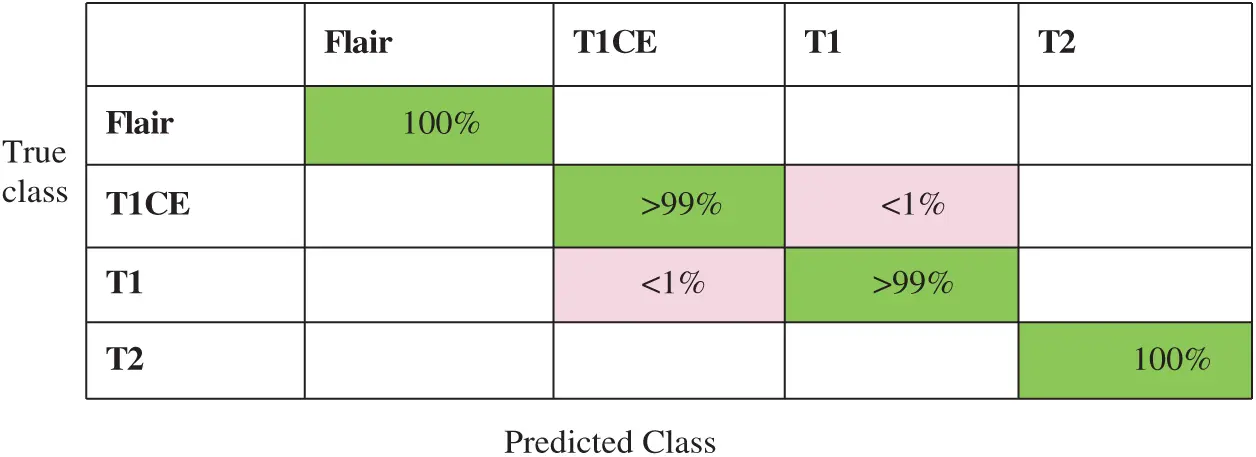Figure 8: Confusion matric of MSVM after employing optimal feature selection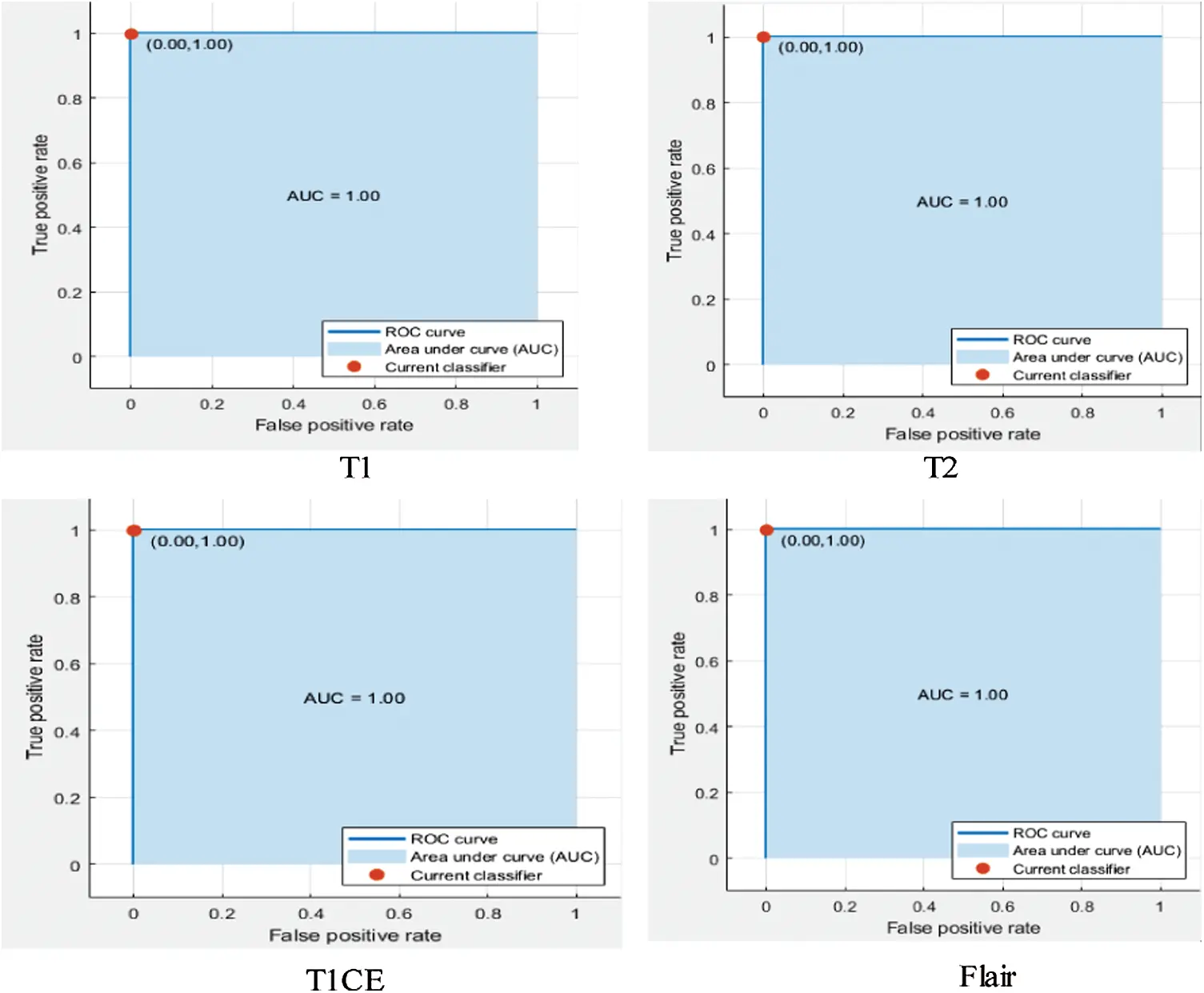Figure 9: ROC plots of MSVM for the verification of AUC

3.2 Testing Results of LGG Images Data

The original feature vectors for the LGG images were fused (Tab. 3). The highest accuracy (99.1%) was achieved by the MSVM, with a sensitivity of 99.00%, precision of 99.00%, F1-score of 99.00%, FPR of 0.002, and AUC of 1.00. The other accuracies were as follows: fine tree, 78.30%; SVM, 93.40%; coarse Gaussian, 82.60%; fine KNN, 98.00%; medium KNN, 91.90%; cubic KNN, 91.90%; weighted KNN, 96.50%; ensemble-boosted tree, 87.10%; and ensemble-bagged tree, 94.10%. In the confusion matrix shown in Fig. 10; the accuracy rate always exceeded 99%. The computational times are listed in Tab. 3 (last column). The fine KNN had the shortest computational time (27.56 s), but the accuracy was only 98.00%, i.e., less than that of the MSVM. The longest computational time was 356.66 s. The MSVM ROC curves are provided in Fig. 11.

Table 3: Classification results of LGG by employing a fused feature vector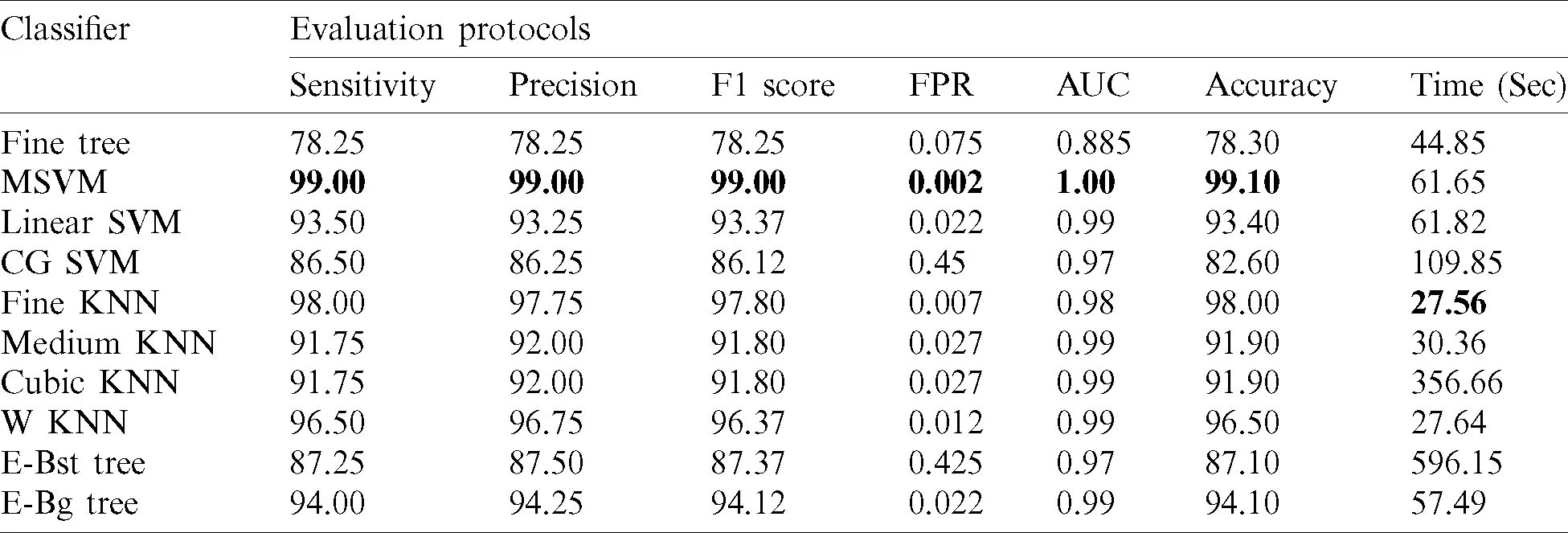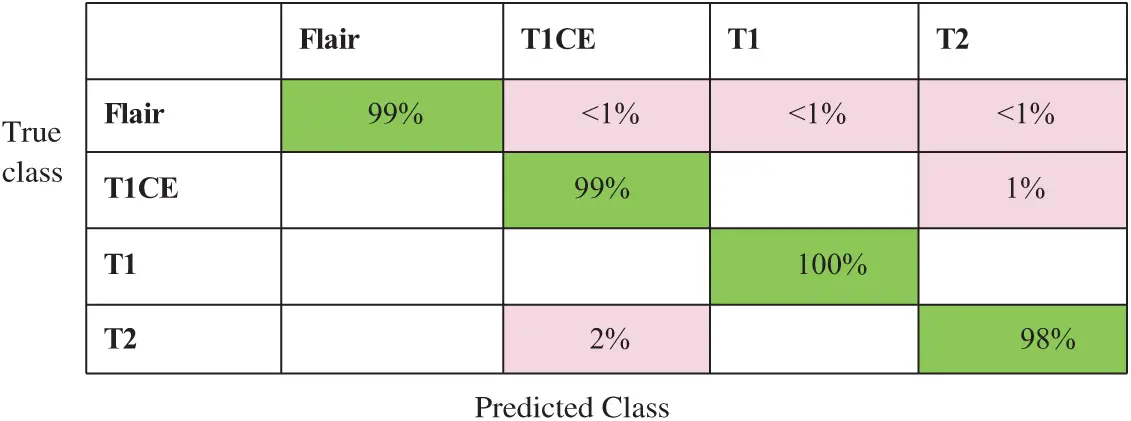Figure 10: Confusion matric of MSVM using fused feature vector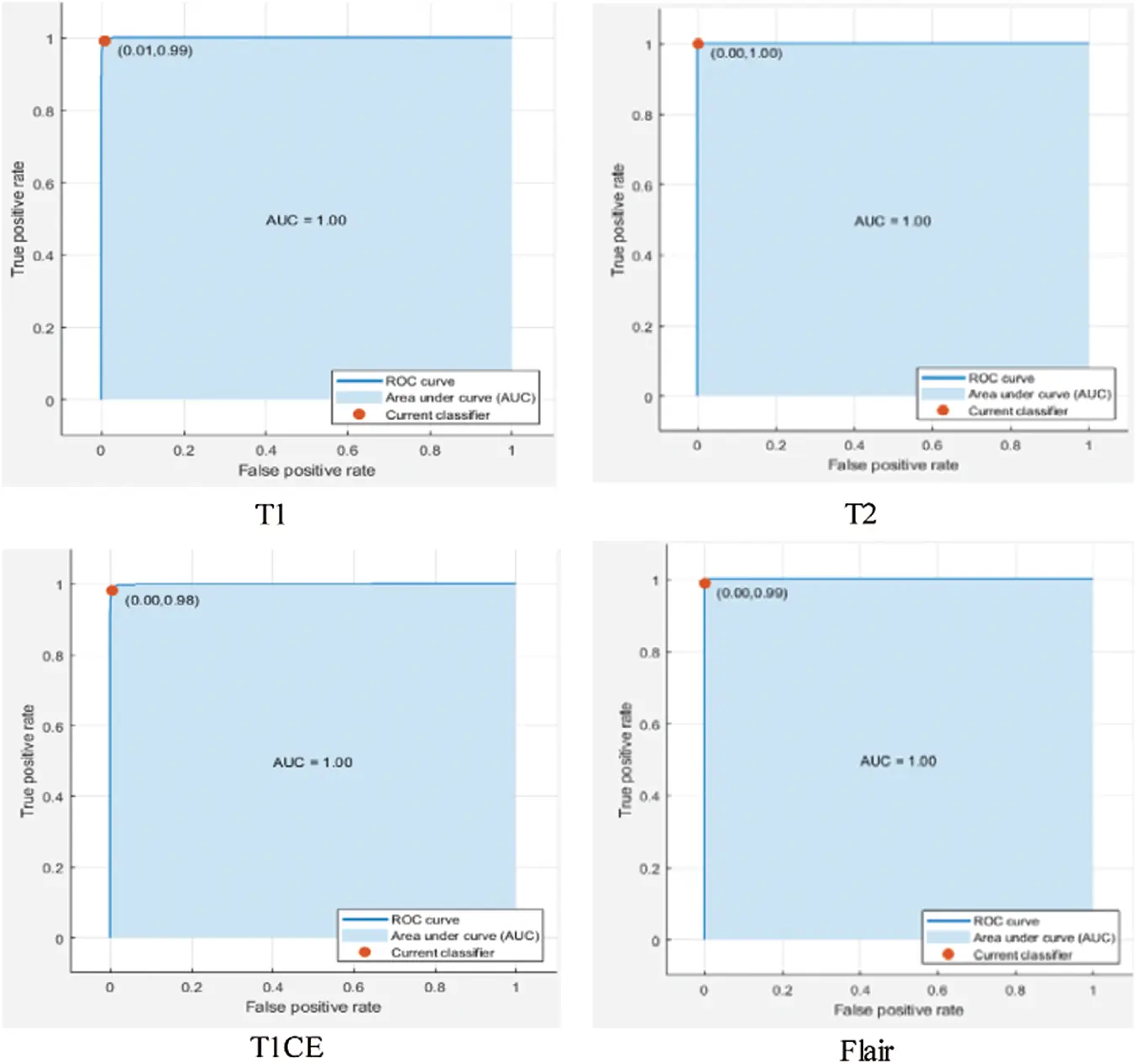Figure 11: ROC plots of MSVM using fused feature vector

The optimized LGG features are listed in Tab. 4. The MSVM showed the best classification performance, with an accuracy of 99.3%, sensitivity of 99.25%, precision of 99.25%, F1-score of 99.25%, FPR of 0.000, and AUC of 1.00. The computational time required was 11.92 s; however, the best time was in fact 6.25 s. The other accuracies were as follows: fine tree, 78.00%; linear SVM, 93.30%; coarse Gaussian, 85.40%; fine KNN, 98.20%; medium KNN, 93.30%; cubic KNN, 93.20%; weighted KNN, 97.30%; ensemble-boosted tree, 83.90%; and ensemble-bagged tree, 93.90%. The confusion matrix is illustrated in Fig. 12; the accuracy rate always exceeded 99%. The MSVM ROC curves are shown in Fig. 13. The use of optimal selected features improved classification accuracy and significantly reduced computational times.

Table 4: Classification results of the proposed method of LGG data after optimal feature selection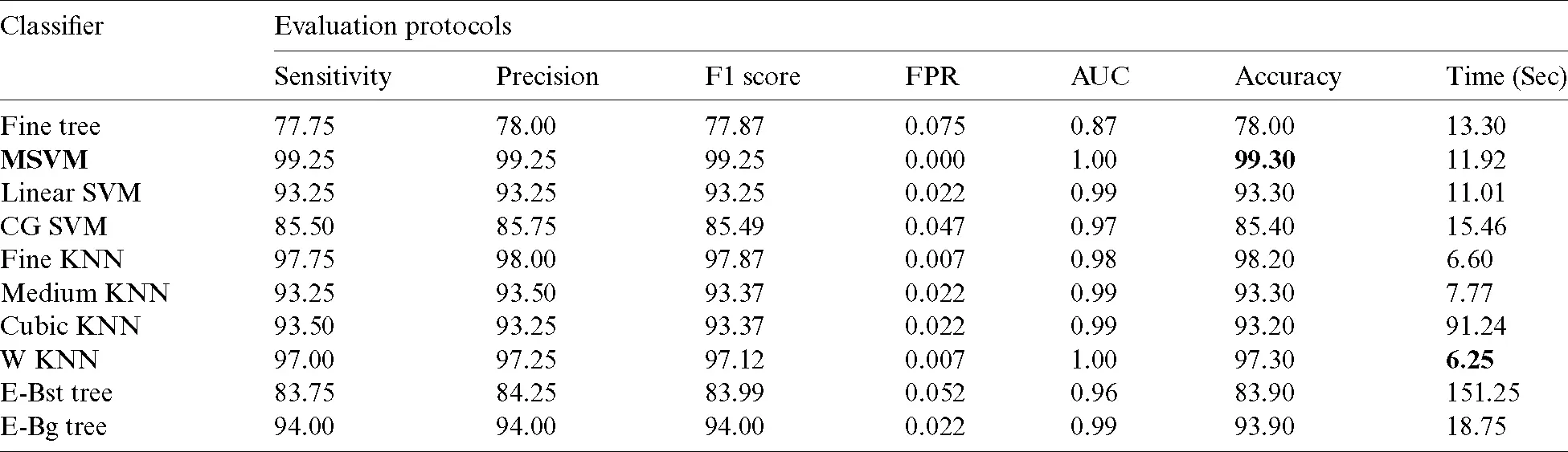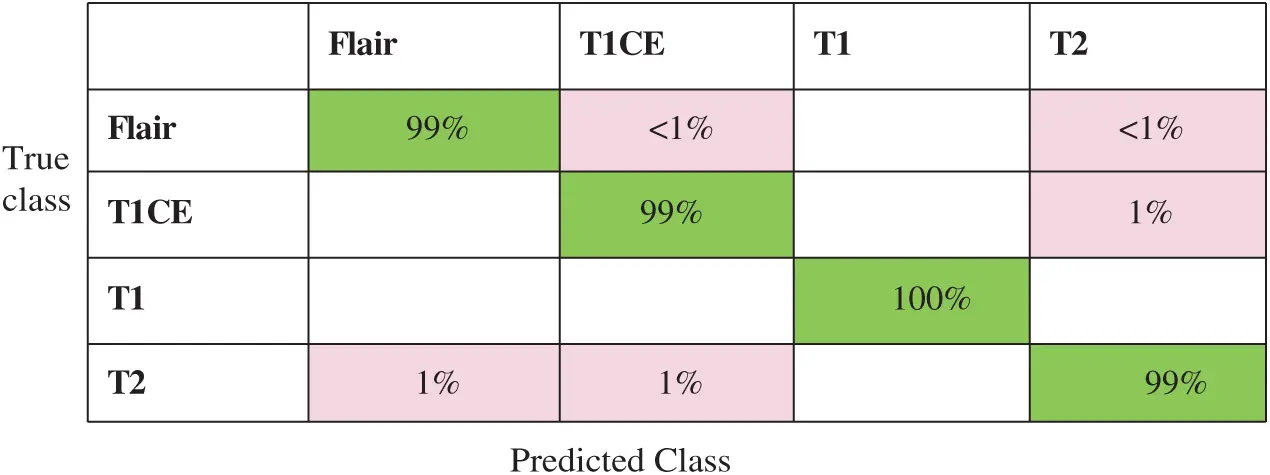Figure 12: Confusion matrix of MSVM on LGG data after employing optimal features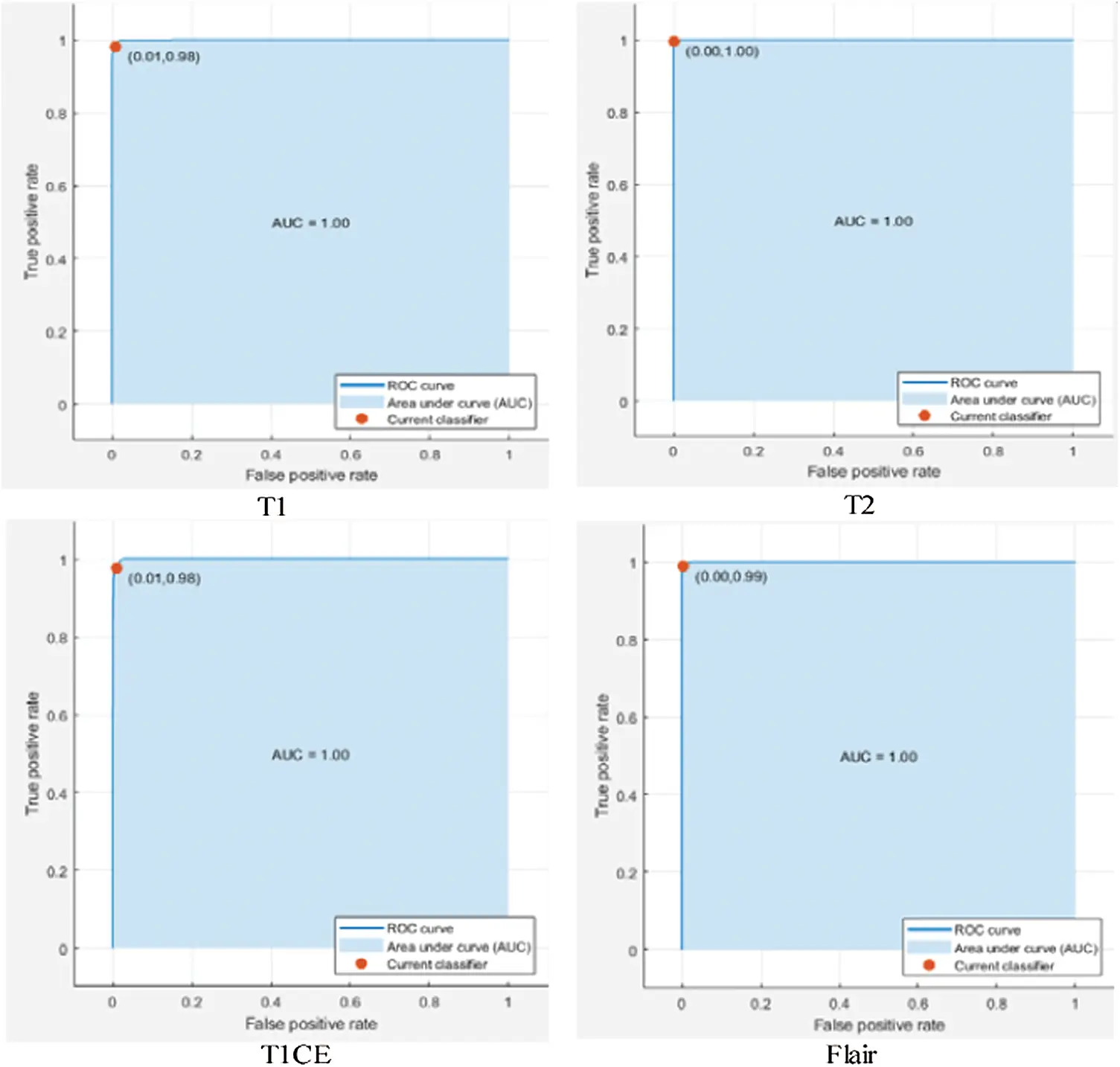Figure 13: Confusion matrix of MSVM on LGG data after employing optimal features

3.3 Comparison with Existing Techniques

Comparison with the existing techniques is also conducted to validate the proposed method (can be seen in Tab. 5). This table shows that the best accuracy previously achieved on the Brats2018 dataset was 98% . In that approach, the authors used the LSTM approach. Amin et al.  achieved the second-best accuracy of 93.85%. In more recent work, Khan et al.  achieved an accuracy of 92.5% using a deep learning framework. Our proposed method is also deep learning-based. We have tested on both HGG and LGG brain images and achieved an accuracy of 99.9% and 99.3%, respectively. The main strength of this work is the selection of the optimal features using an improved PSO algorithm. Moreover, the proposed labeled results are also given in Fig. 14.

Table 5: Comparison of the proposed method results with existing techniques for the BRATS2018 dataset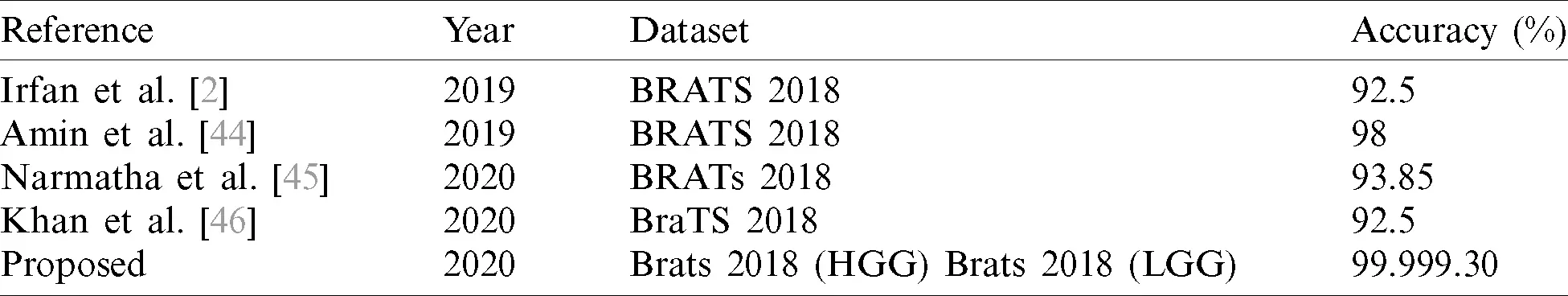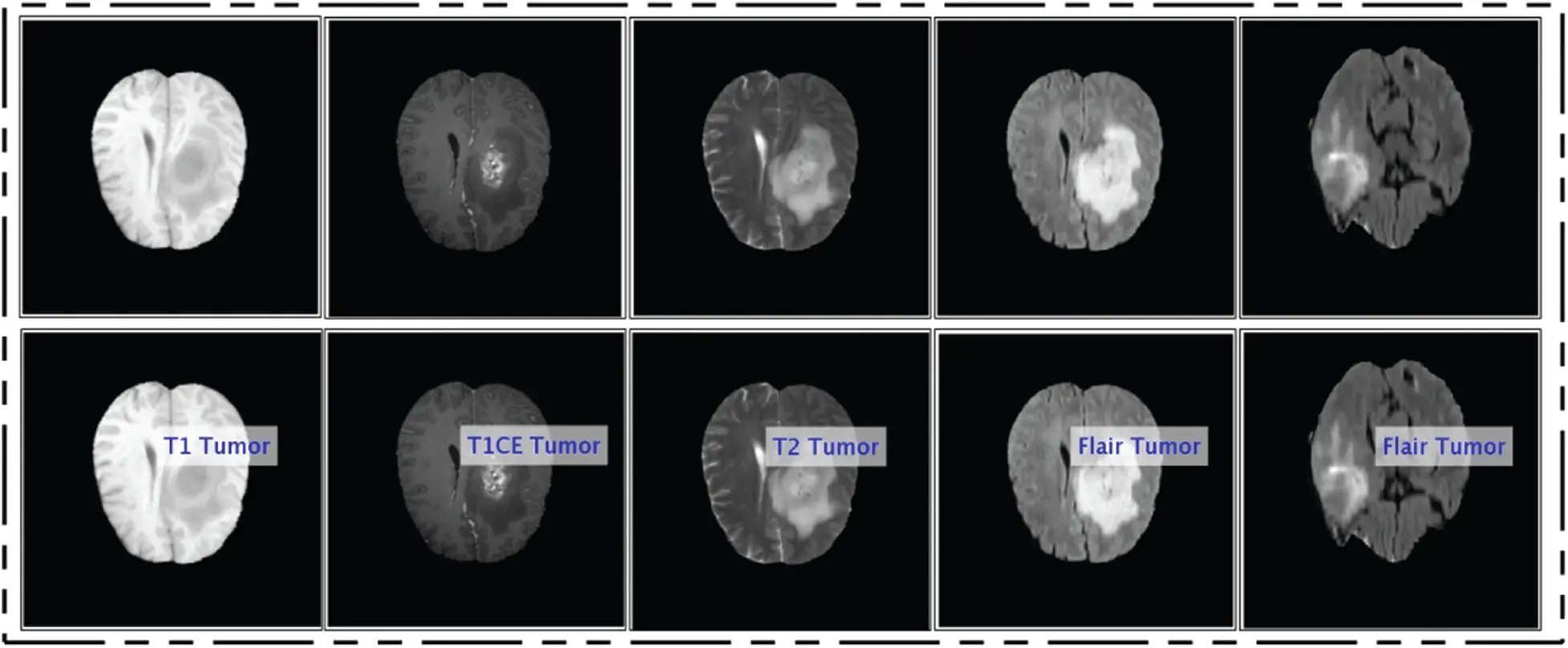Figure 14: Prediction results of the proposed method in the form of corresponding labels

4  Conclusion

A new automated technique is proposed in this article for brain tumor classification using deep learning and the IPSO algorithm. The contrast of original MRI scans is enhanced using the ACO approach to learn a better CNN model. This step not only enhances the tumor region but also extracts more relevant features. Later, fusion of two-layer features improves the original accuracy of classification. A few redundant features are also added in the fusion process for classification, which does not yield the target accuracy. Therefore, another algorithm called the IPSO is proposed to improve the system’s accuracy and minimize computational time. Hence, we conclude that the most optimum features give better classification accuracy and decrease the system prediction time. The major limitation of this work is the proposed stopping criterion. There is a chance that the features after the stopping condition may perform well. In future, we aim to try to enhance this stopping criterion and will perform experiments on the BraTs2019 dataset as well.

Funding Statement: This research was supported by Korea Institute for Advancement of Technology (KIAT) grant funded by the Korea Government (MOTIE) (P0012724, The Competency Development Program for Industry Specialist) and the Soonchunhyang University Research Fund.

Conflicts of Interest: The authors declare that they have no conflicts of interest to report regarding the present study.

## References

1. A. Tiwari, S. Srivastava and M. Pant. (2019). “Brain tumor segmentation and classification from magnetic resonance images: Review of selected methods from 2014 to 2019,” Pattern Recognition Letters, vol. 131, no. 9, pp. 244–260.
2. M. I. Sharif, J. P. Li, M. A. Khan and M. A. Saleem. (2020). “Active deep neural network features selection for segmentation and recognition of brain tumors using MRI images,” Pattern Recognition Letters, vol. 129, no. 10, pp. 181–189.
3. J. Amin, M. Sharif, N. Gul, M. Yasmin and S. A. Shad. (2020). “Brain tumor classification based on DWT fusion of MRI sequences using convolutional neural network,” Pattern Recognition Letters, vol. 129, pp. 115–122.
4. M. Sharif, J. Amin, M. Raza, M. A. Anjum, H. Afzal et al. (2020). , “Brain tumor detection based on extreme learning,” Neural Computing and Applications, vol. 32, no. 20, pp. 15975–15987.
5. A. M. Molinaro, S. Hervey-Jumper, R. A. Morshed, J. Young, S. J. Han et al. (2020). , “Association of maximal extent of resection of contrast-enhanced and non-contrast-enhanced tumor with survival within molecular subgroups of patients with newly diagnosed glioblastoma,” JAMA Oncology, vol. 4, no. 4, pp. 495–503.
6. Y. D. Zhang, Z. Dong, S. H. Wang, X. Yu, X. Yao et al. (2020). , “Advances in multimodal data fusion in neuroimaging: Overview, challenges, and novel orientation,” Information Fusion, vol. 64, no. Suppl 3, pp. 149–187.
7. Y. D. Zhang, S. C. Satapathy, S. Liu and G. R. Li. (2020). “A five-layer deep convolutional neural network with stochastic pooling for chest CT-based COVID-19 diagnosis,” Machine Vision and Applications, vol. 32, no. 1, pp. 1–13.
8. M. A. Khan, S. Kadry, M. Alhaisoni, Y. Nam, Y. Zhang et al. (2020). , “Computer-aided gastrointestinal diseases analysis from wireless capsule endoscopy: A framework of best features selection,” IEEE Access, vol. 8, pp. 132850–132859.
9. M. A. Khan, I. U. Lali, A. Rehman, M. Ishaq, M. Sharif et al. (2019). , “Brain tumor detection and classification: A framework of marker-based watershed algorithm and multilevel priority features selection,” Microscopy Research and Technique, vol. 82, no. 6, pp. 909–922.
10. S. H. Wang, V. V. Govindaraj, J. M. Górriz, X. Zhang and Y. D. Zhang. (2020). “Covid-19 classification by FGCNet with deep feature fusion from graph convolutional network and convolutional neural network,” Information Fusion, vol. 67, pp. 208–229.
11. S. H. Wang and Y. D. Zhang. (2020). “DenseNet-201-based deep neural network with composite learning factor and precomputation for multiple sclerosis classification,” ACM Transactions on Multimedia Computing, Communications, and Applications, vol. 16, pp. 1–19.
12. U. Nazar, M. A. Khan, I. U. Lali, H. Lin, H. Ali et al. (2020). , “Review of automated computerized methods for brain tumor segmentation and classification,” Current Medical Imaging, vol. 16, no. 7, pp. 823–834.
13. B. Kaur, M. Sharma, M. Mittal, A. Verma, L. M. Goyal et al. (2018). , “An improved salient object detection algorithm combining background and foreground connectivity for brain image analysis,” Computers & Electrical Engineering, vol. 71, no. 11, pp. 692–703.
14. M. A. Khan, M. Sharif, T. Akram, S. A. C. Bukhari and R. S. Nayak. (2020). “Developed Newton–Raphson based deep features selection framework for skin lesion recognition,” Pattern Recognition Letters, vol. 129, no. 4/5, pp. 293–303.
15. M. A. Khan, S. Rubab, A. Kashif, M. I. Sharif, N. Muhammad et al. (2020). , “Lungs cancer classification from CT images: An integrated design of contrast based classical features fusion and selection,” Pattern Recognition Letters, vol. 129, pp. 77–85.
16. M. A. Khan, M. S. Sarfraz, M. Alhaisoni, A. A. Albesher, S. Wang et al. (2020). , “StomachNet: Optimal deep learning features fusion for stomach abnormalities classification,” IEEE Access, vol. 8, pp. 197969– 197981.
17. D. J. Hemanth, J. Anitha and M. Mittal. (2018). “Diabetic retinopathy diagnosis from retinal images using modified hopfield neural network,” Journal of Medical Systems, vol. 42, no. 12, pp. 247.
18. M. A. Khan, M. Qasim, H. M. J. Lodhi, M. Nazir, K. Javed et al. (2020). , “Automated design for recognition of blood cells diseases from hematopathology using classical features selection and ELM,” Microscopy Research and Technique, vol. 2, pp. 1–21.
19. M. A. Khan, M. A. Khan, F. Ahmed, M. Mittal, L. M. Goyal et al. (2020). , “Gastrointestinal diseases segmentation and classification based on duo-deep architectures,” Pattern Recognition Letters, vol. 131, pp. 193–204.
20. A. Mittal, D. Kumar, M. Mittal, T. Saba, I. Abunadi et al. (2020). , “Detecting pneumonia using convolutions and dynamic capsule routing for chest x-ray images,” Sensors, vol. 20, no. 4, pp. 1068.
21. S. Dash, B. R. Acharya, M. Mittal, A. Abraham and A. Kelemen. (2020). “Deep learning techniques for biomedical and health informatics,” in Studies in Big Data. vol. 68. Cham: Springer.
22. M. Mittal, L. M. Goyal, S. Kaur, I. Kaur, A. Verma et al. (2019). , “Deep learning based enhanced tumor segmentation approach for MR brain images,” Applied Soft Computing, vol. 78, no. 10, pp. 346–354.
23. D. Abirami, N. Shalini, V. Rajinikanth, H. Lin and V. S. Rao. (2020). “Brain MRI examination with varied modality fusion and chan-vese segmentation,” in Intelligent Data Engineering and Analytics. Cham: Springer, pp. 671–679.
24. V. Rajinikanth, A. N. Joseph Raj, K. P. Thanaraj and G. R. Naik. (2020). “A customized VGG19 network with concatenation of deep and handcrafted features for brain tumor detection,” Applied Sciences, vol. 10, no. 10, pp. 3429.
25. R. Pugalenthi, M. Rajakumar, J. Ramya and V. Rajinikanth. (2019). “Evaluation and classification of the brain tumor MRI using machine learning technique,” Journal of Control Engineering and Applied Informatics, vol. 21, pp. 12–21.
26. S. L. Fernandes, U. J. Tanik, V. Rajinikanth and K. A. Karthik. (2020). “A reliable framework for accurate brain image examination and treatment planning based on early diagnosis support for clinicians,” Neural Computing and Applications, vol. 32, no. 20, pp. 15897–15908.
27. N. Arunkumar, M. A. Mohammed, S. A. Mostafa, D. A. Ibrahim, J. J. Rodrigues et al. (2020). , “Fully automatic model-based segmentation and classification approach for MRI brain tumor using artificial neural networks,” Concurrency and Computation: Practice and Experience, vol. 32, no. 1, pp. e4962.
28. N. Arunkumar, M. A. Mohammed, M. K. Abd Ghani, D. A. Ibrahim, E. Abdulhay et al. (2019). , “K-means clustering and neural network for object detecting and identifying abnormality of brain tumor,” Soft Computing, vol. 23, no. 19, pp. 9083–9096.
29. M. K. Abd Ghani, M. A. Mohammed, N. Arunkumar, S. A. Mostafa, D. A. Ibrahim et al. (2020). , “Decision-level fusion scheme for nasopharyngeal carcinoma identification using machine learning techniques,” Neural Computing and Applications, vol. 32, no. 3, pp. 625–638.
30. O. I. Obaid, M. A. Mohammed, M. Ghani, A. Mostafa and F. Taha. (2018). “Evaluating the performance of machine learning techniques in the classification of wisconsin breast cancer,” International Journal of Engineering & Technology, vol. 7, pp. 160–166.
31. M. A. Mohammed, K. H. Abdulkareem, S. A. Mostafa, M. K. A. Ghani, M. S. Maashi et al. (2020). , “Voice pathology detection and classification using convolutional neural network model,” Applied Sciences, vol. 10, no. 11, pp. 3723.
32. T. Saba, A. S. Mohamed, M. El-Affendi, J. Amin and M. Sharif. (2020). “Brain tumor detection using fusion of hand crafted and deep learning features,” Cognitive Systems Research, vol. 59, no. 1, pp. 221–230.
33. J. Sachdeva, V. Kumar, I. Gupta, N. Khandelwal and C. K. Ahuja. (2013). “Segmentation, feature extraction, and multiclass brain tumor classification,” Journal of Digital Imaging, vol. 26, no. 6, pp. 1141–1150.
34. H. Mohsen, E. S. A. El-Dahshan, E. S. M. El-Horbaty and A. B. M. Salem. (2018). “Classification using deep learning neural networks for brain tumors,” Future Computing and Informatics Journal, vol. 3, no. 1, pp. 68–71.
35. U. N. Hussain, M. A. Khan, I. U. Lali, K. Javed, I. Ashraf et al. (2020). , “A unified design of ACO and skewness based brain tumor segmentation and classification from MRI scans,” Journal of Control Engineering and Applied Informatics, vol. 22, pp. 43–55.
36. M. Rashid, M. A. Khan, M. Alhaisoni, S. H. Wang, S. R. Naqvi et al. (2020). , “A sustainable deep learning framework for object recognition using multi-layers deep features fusion and selection,” Sustainability, vol. 12, no. 12, pp. 5037.
37. W. Rawat and Z. Wang. (2017). “Deep convolutional neural networks for image classification: A comprehensive review,” Neural Computation, vol. 29, no. 9, pp. 2352–2449.
38. S. Dutta, B. Manideep, S. Rai and V. Vijayarajan. (2017). “A comparative study of deep learning models for medical image classification,” IOP Conference Series: Materials Science and Engineering, vol. 263, no. 4, pp. 42097.
39. K. Simonyan and A. Zisserman. (2015). “Very deep convolutional networks for large-scale image recognition,” in Int. Conf. on Learning Representations.
40. K. Weiss, T. M. Khoshgoftaar and D. Wang. (2016). “A survey of transfer learning,” Journal of Big Data, vol. 3, no. 1, pp. 1–9.
41. N. Naheed, M. Shaheen, S. A. Khan, M. Alawairdhi and M. A. Khan. (2020). “Importance of features selection, attributes selection, challenges and future directions for medical imaging data: A review,” Computer Modeling in Engineering & Sciences, vol. 125, pp. 314–344.
42. B. Xue, M. Zhang and W. N. Browne. (2012). “Particle swarm optimization for feature selection in classification: A multi-objective approach,” IEEE Transactions on Cybernetics, vol. 43, no. 6, pp. 1656–1671.
43. M. M. Kabir, M. Shahjahan and K. Murase. (2011). “A new local search based hybrid genetic algorithm for feature selection,” Neurocomputing, vol. 74, no. 17, pp. 2914–2928.
44. L. Weninger, O. Rippel, S. Koppers and D. Merhof. (2018). “Segmentation of brain tumors and patient survival prediction: Methods for the BraTS, 2018 challenge,” in International MICCAI Brainlesion Workshop. Cham: Springer, pp. 3–12.
45. J. Amin, M. Sharif, M. Raza, T. Saba, R. Sial et al. (2019). , “Brain tumor detection: A long short-term memory (LSTM)-based learning model,” Neural Computing and Applications, vol. 32, no. 20, pp. 1–9.
46. C. Narmatha, S. M. Eljack, A. A. R. M. Tuka, S. Manimurugan and M. Mustafa. (2020). “A hybrid fuzzy brain-storm optimization algorithm for the classification of brain tumor MRI images,” Journal of Ambient Intelligence and Humanized Computing, vol. 7, no. 10, pp. 1–9.This work is licensed under a Creative Commons Attribution 4.0 International License, which permits unrestricted use, distribution, and reproduction in any medium, provided the original work is properly cited.